# Methods and formulas for fitted means in Fit Mixed Effects Model

Select the method or formula of your choice.

## Conditional Fitted Value

Suppose a model has two factors, A and B and the interaction between the 2 factors, A*B. A is a random factor and B is a fixed factor.

The following equation represents the response value at level i of factor A and level j of factor B:

where

•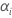is for the random factor A,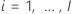•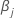is for the fixed factor B,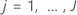•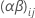is for the AB term
•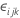is for the error term

The equation for the conditional fitted value for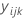follows:

where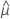and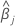represent the corresponding calculated coefficients for the fixed effect terms. Also,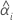and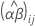are the calculated BLUP values for the random terms.

## Mean of AB

The calculated mean of AB at level i of factor A and level j of factor B is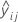.

## Mean of A

The calculated mean of A at level i follows:

## Mean of B

The calculated mean of B at level j follows:

## General formula for means

In general, any mean can be represented by the following vector expression:

where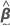is the calculated coefficient vector,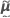is the calculated BLUP vector, and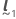and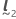are known vectors determined by the mean for a specific term. Then the mean formula has the same form as the conditional fit. Therefore, the formulas for standard error, the degrees of freedom, the confidence interval, the t-value, and the p-value for the mean are simlilarly derived as the corresponding formulas for the conditional fits. For more information on these formulas for the conditional fits, go to Methods and formulas for conditional fits and residuals in Fit Mixed Effects Model. By replacing the vector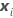and the vector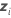with the vectorand the vectorrespectively in the conditional fit formulas, you get the corresponding formulas for the mean.

By using this site you agree to the use of cookies for analytics and personalized content.  Read our policy